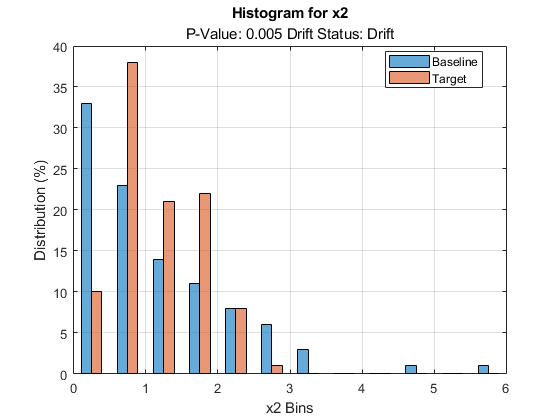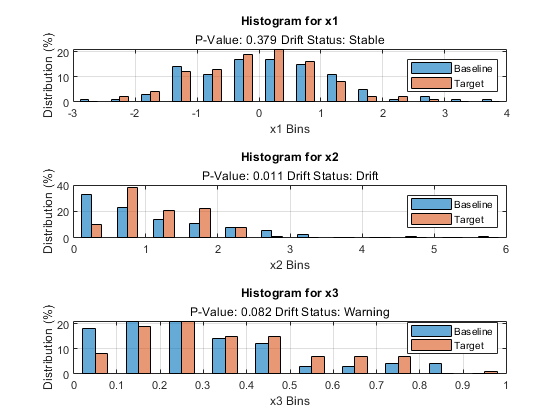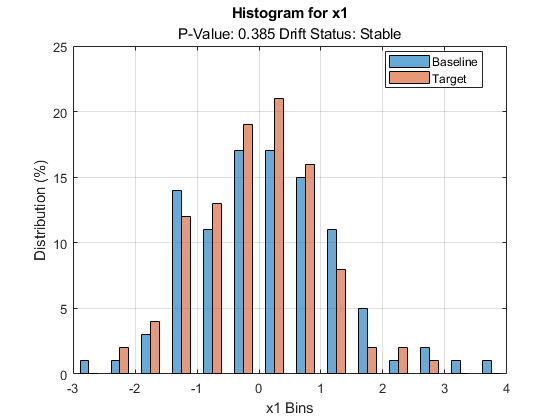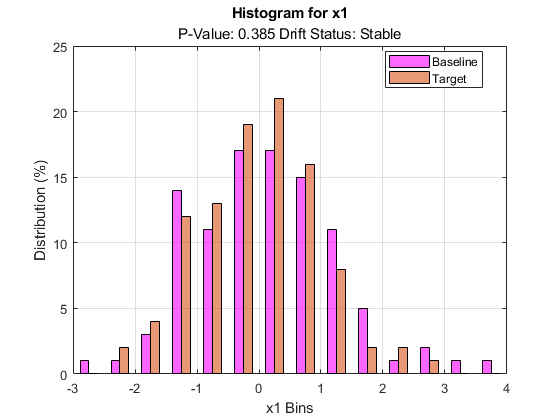# plotHistogram

Plot histogram of a variable specified for data drift detection

Since R2022a

## Syntax

``plotHistogram(DDiagnostics)``
``plotHistogram(DDiagnostics,Variable=variable)``
``plotHistogram(ax,___)``
``H = plotHistogram(___)``

## Description

example

````plotHistogram(DDiagnostics)` plots a histogram of the baseline and target data for the variable with the lowest p-value computed by the `detectdrift` function.If you set the value of `EstimatePValues` to `false` in the call to `detectdrift`, then `plotHistogram` displays `NaN` for the p-value and the drift status.```
````plotHistogram(DDiagnostics,Variable=variable)` plots the histogram of the baseline and target data for the variable specified by `variable`.```

example

````plotHistogram(ax,___)` plots on the axes `ax` instead of `gca`, using any of the input argument combinations in the previous syntaxes.```

example

````H = plotHistogram(___)` plots the histogram and returns an array of `Histogram` objects in `H`. Use `H` to inspect and modify the properties of the histogram. For more information, see Histogram Properties.```

## Examples

collapse all

Generate baseline and target data with three variables, where the distribution parameters of the second and third variables change for the target data.

```rng('default') % For reproducibility baseline = [normrnd(0,1,100,1),wblrnd(1.1,1,100,1),betarnd(1,2,100,1)]; target = [normrnd(0,1,100,1),wblrnd(1.2,2,100,1),betarnd(1.7,2.8,100,1)];```

Perform permutation testing for all variables to check for any drift between the baseline and target data.

`DDiagnostics = detectdrift(baseline,target)`
```DDiagnostics = DriftDiagnostics VariableNames: ["x1" "x2" "x3"] CategoricalVariables: [] DriftStatus: ["Stable" "Drift" "Warning"] PValues: [0.3850 0.0050 0.0910] ConfidenceIntervals: [2×3 double] MultipleTestDriftStatus: "Drift" DriftThreshold: 0.0500 WarningThreshold: 0.1000 Properties, Methods ```

Plot the histogram for the default variable.

`plotHistogram(DDiagnostics)`By default, `plotHistogram` plots a histogram of the baseline and target data for the variable with the lowest p-value. The function also displays the p-value and the drift status for the variable.

Generate baseline and target data with three variables, where the distribution parameters of the second and third variables change for the target data.

```rng('default') % For reproducibility baseline = [normrnd(0,1,100,1),wblrnd(1.1,1,100,1),betarnd(1,2,100,1)]; target = [normrnd(0,1,100,1),wblrnd(1.2,2,100,1),betarnd(1.7,2.8,100,1)];```

Perform permutation testing for all variables to check for any drift between the baseline and target data. Use the Energy statistic as the metric.

`DDiagnostics = detectdrift(baseline,target,ContinuousMetric="energy")`
```DDiagnostics = DriftDiagnostics VariableNames: ["x1" "x2" "x3"] CategoricalVariables: [] DriftStatus: ["Stable" "Drift" "Warning"] PValues: [0.3790 0.0110 0.0820] ConfidenceIntervals: [2×3 double] MultipleTestDriftStatus: "Drift" DriftThreshold: 0.0500 WarningThreshold: 0.1000 Properties, Methods ```

Plot the histograms for all three variables in a tiled layout.

```tiledlayout(3,1); ax1 = nexttile; plotHistogram(DDiagnostics,ax1,Variable="x1") ax2 = nexttile; plotHistogram(DDiagnostics,ax2,Variable="x2") ax3 = nexttile; plotHistogram(DDiagnostics,ax3,Variable="x3")```Generate baseline and target data with three variables, where the distribution parameters of the second and third variables change for the target data.

```rng('default') % For reproducibility baseline = [normrnd(0,1,100,1),wblrnd(1.1,1,100,1),betarnd(1,2,100,1)]; target = [normrnd(0,1,100,1),wblrnd(1.2,2,100,1),betarnd(1.7,2.8,100,1)];```

Perform permutation testing for all variables to check for any drift between the baseline and target data.

`DDiagnostics = detectdrift(baseline,target)`
```DDiagnostics = DriftDiagnostics VariableNames: ["x1" "x2" "x3"] CategoricalVariables: [] DriftStatus: ["Stable" "Drift" "Warning"] PValues: [0.3850 0.0050 0.0910] ConfidenceIntervals: [2×3 double] MultipleTestDriftStatus: "Drift" DriftThreshold: 0.0500 WarningThreshold: 0.1000 Properties, Methods ```

Plot the histogram for the first variable and return the `Histogram` object.

`H = plotHistogram(DDiagnostics,Variable=1)````H = 2×1 Bar array: Bar (Baseline) Bar (Target) ```

Change the color of the histogram bars for the baseline data.

`H(1).FaceColor = [1 0 1];`## Input Arguments

collapse all

Diagnostics of the permutation testing for drift detection, specified as a `DriftDiagnostics` object returned by `detectdrift`.

Variable for which to plot the histogram, specified as a string, a character vector, or an integer index.

Example: `Variable="x2"`

Example: `Variable=2`

Data Types: `single` | `double` | `char` | `string`

Axes for `plotHistogram` to plot into, specified as an `Axes` or `UIAxes` object. If you do not specify `ax`, then `plotHistogram` creates the plot using the current axes. For more information on creating an axes object, see `axes` and `uiaxes`.

## Algorithms

• For categorical data, `detectdrift` adds a 0.5 correction factor to the histogram bin counts for each bin to handle empty bins (categories). This is equivalent to the assumption that the parameter p, probability that value of the variable would be in that category, has the prior distribution Beta(0.5,0.5), (Jeffreys prior assumption for the distribution parameter).

• `plotHistogram` treats a variable as ordinal for visualization purposes in these cases:

• The variable is ordinal in either the baseline data or the target data, and the categories from both the baseline data and the target data are the same.

• The variable is ordinal in either the baseline data or the target data, and the categories of the other data set are a subset of the ordinal data.

• The variable is ordinal in both the baseline data and the target data, and categories from either data set are a subset of the other.

• If a variable is ordinal, `plotHistogram` preserves the order of the bin names.

## Version History

Introduced in R2022a